xMethod of undetermined coefficientsEncyclopedia
In mathematics
Mathematics
Mathematics is the study of quantity, space, structure, and change. Mathematicians seek out patterns and formulate new conjectures. Mathematicians resolve the truth or falsity of conjectures by mathematical proofs, which are arguments sufficient to convince other mathematicians of their validity...

, the method of undetermined coefficients, also known as the lucky guess method, is an approach to finding a particular solution to certain inhomogeneous ordinary differential equation
Ordinary differential equation
In mathematics, an ordinary differential equation is a relation that contains functions of only one independent variable, and one or more of their derivatives with respect to that variable....

s and recurrence relation
Recurrence relation
In mathematics, a recurrence relation is an equation that recursively defines a sequence, once one or more initial terms are given: each further term of the sequence is defined as a function of the preceding terms....

s. It is closely related to the annihilator method
Annihilator method
In mathematics, the annihilator method is a procedure used to find a particular solution to certain types of inhomogeneous ordinary differential equations. It is similar to the method of undetermined coefficients, but instead of guessing the particular solution in the method of undetermined...

, but instead of using a particular kind of differential operator (the annihilator) in order to find the best possible form of the particular solution, a "guess" is made as to the appropriate form, which is then tested by differentiating the resulting equation. For complex equations, the annihilator method or variation of parameters is less time consuming to perform.

Undetermined coefficients is not as general a method as variation of parameters, since it only works for differential equations that follow certain forms.

## Description of the method

Consider a linear non-homogeneous ordinary differential equation of the form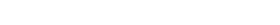The method consists of finding the general homogeneous
Homogeneous differential equation
The term homogeneous differential equation has several distinct meanings.One meaning is that a first-order ordinary differential equation is homogeneous if it has the formwhere F is a homogeneous function of degree zero; that is to say, that F = F.In a related, but distinct, usage, the term linear...

solution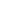for the complementary linear homogeneous differential equation
Homogeneous differential equation
The term homogeneous differential equation has several distinct meanings.One meaning is that a first-order ordinary differential equation is homogeneous if it has the formwhere F is a homogeneous function of degree zero; that is to say, that F = F.In a related, but distinct, usage, the term linear...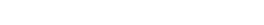and a particular integral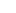of the linear non-homogeneous ordinary differential equation based on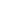. Then the general solution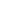to the linear non-homogeneous ordinary differential equation would be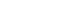Ifconsists of the sum of two functions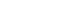and we say that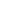is the solution based on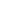and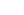the solution based on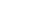. Then, using a superposition principle
Superposition principle
In physics and systems theory, the superposition principle , also known as superposition property, states that, for all linear systems, the net response at a given place and time caused by two or more stimuli is the sum of the responses which would have been caused by each stimulus individually...

, we can say that the particular integralis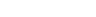## Typical forms of the particular integral

In order to find the particular integral, we need to 'guess' its form, with some coefficients left as variables to be solved for. This takes the form of the first derivative of complementary function. Below is a table of some typical functions and the solution to guess for them.

 Function of x Form for y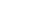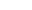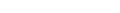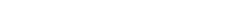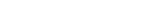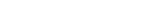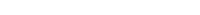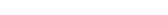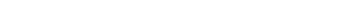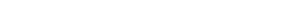If a term in the above particular integral for y appears in the homogeneous solution, it is necessary to multiply by a sufficiently large power of x in order to make the two solutions linearly independent. If the function of x is a sum of terms in the above table, the particular integral can be guessed using a sum of the corresponding terms for y.

## Examples

Example 1

Find a particular integral of the equation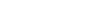The right side t cos t has the form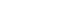with n=1, α=0, and β=1.

Since α + iβ = i is a simple root of the characteristic equation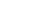we should try a particular integral of the formSubstituting yp into the differential equation, we have the identityComparing both sides, we have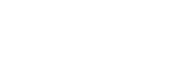which has the solution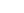= 0,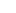= 1/4,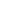= 1/4,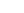= 0. We then have a particular integral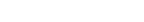Example 2

Consider the following linear inhomogeneous differential equation: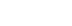This is like the first example above, except that the inhomogeneous part (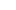) is not linearly independent to the general solution of the homogeneous part (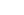); as a result, we have to multiply our guess by a sufficiently large power of x to make it linearly independent.

Here our guess becomes: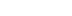By substituting this function and its derivative into the differential equation, one can solve for A: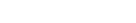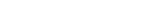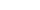So, the general solution to this differential equation is thus: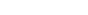Example 3

Find the general solution of the equation: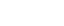f(t),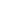, is a polynomial of degree 2, so we look for a solution using the same form,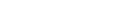, where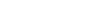Plugging this particular integral with constants A, B, and C into the original equation yields,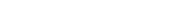, where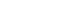andandReplacing resulting constants,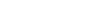To solve for the general solution,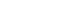whereis the homogeneous solution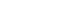,
therefore, the general solution is: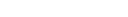The source of this article is wikipedia, the free encyclopedia.  The text of this article is licensed under the GFDL.